# 1st PUC Computer Science Previous Year Question Paper March 2016 (South)

Students can Download 1st PUC Computer Science Previous Year Question Paper March 2016 (South), Karnataka 1st PUC Computer Science Model Question Papers with Answers helps you to revise the complete Karnataka State Board Syllabus and score more marks in your examinations.

## Karnataka 1st PUC Computer Science Previous Year Question Paper March 2016 (South)

Time: 3 Hours 15 Minutes
Max. Marks: 100

PART – A

I. Answer the following questions. (10 × 1 = 10)

a

Question 1.
Which is the earliest computing device?
Abacus is the oldest computing tool.

Question 2.
Expand OCR.

Question 3.
What is testing?
It is the process of checking the program logic, by providing selected sample data and observing the output for correctness.

Question 4.
Define token in C++.
The smallest individual units in a program are known as tokens, on lexical units.

Question 5.
Name the stream insertion operator.
cout is used in conjuction with << operator, known as insertion or put to operator.

Question 6.
What is selection statement?
The Selection statements are used to execute certain block of statements after evaluating the condition.

Question 7.
How do you initialize one dimensional array?
intmarks = { 91,96,90,94,99,93};

Question 8.
What are local variables?
The variables declared within a function block are called local variables.Question 9.
Define mail merge.
Mail merge is a process helps to generate letters by combing contents of one document with the content of addresses.

Question 10.
What is a cell in ESS?
The intersection of a row and a column in a spread sheet is called a cell.

PART-B

II. Answer any Five of the following questions. Each question carries two marks: (5 × 2 = 10)

Question 11.
Write a note on pascaline.

Question 12.
Give the difference between hardcopy and softcopy.

1. A hard copy is a copy of text, image or sound that has been printed on paper. Hard copies allow data to be read without the need of a computer.
2. A soft copy is a copy of text, image or sound stored on the computer and only accessible through the computer

Question 13.
Mention the types of software.
The system software, Application software, utility programs are the different types of software.

Question 14. Write different types of programming constructs.

• Sequence of sequentially executed statements.
• Conditional execution of statements.
• Iteration execution statements

Question 15.
Write any two characteristics of OOPs.
Data encapsulation and inheritance are two important characteristics of OOP.

Question 16.
What is data type? Give an example.
The set of values along with the operations that can be performed on these values are called data types.
Example:
intQuestion 17.
How do you initialize a string? Give an example.
char name=”bharath”;

Question 18.
Give any two word processor software.
The two word processor software are MS word and Winword

PART-C

III. Answer any Five of the following Questions. Each question carries three marks: (5 × 3 = 15)

Question 19.
Name any three secondary storage devices.
The three secondary storage devices are Hard Disks, Magnetic Tapes and Floppy disks, Pen drivesetc.,

Question 20.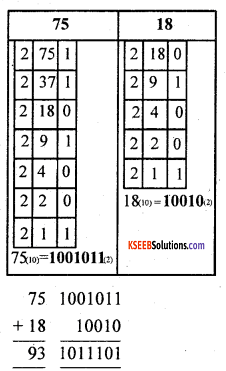Question 21.
Give any three types of operating systems.
Single user operating system, Batch operating system, Distributed operating system and network operating system

Question 22.
Mention different types of errors.
The different program errors are as follows:
1. Syntax error:
It occurs when there is a violation in the grammatical rules of a programming language’s instructions. It happens at the time of compilation. Such errors need to be rectified before proceeding further.

2. Semantic errors:
An error, which occurs due to the incorrect logic in a solution is called semantic error. It also occurs due to wrong use of grammar in the program?

3. Runtime Error:
It occur at run-time. Such error cause a program to end abruptly or even cause system shut-down. Such errors are hard to detect and are known as ‘Bugs’

4. Logical Error :
It may happen that a program contains no syntax or run-time errors but still it doesn’t produce the correct output. It is because the developer has not understood the problem statement properly. These errors are hard to detect as well. It may need the algonithm to be modified in the design phase and changing sources code.Question 23.
Explain logical operators.
Logical operators are used to combine one or more relational expressions. Examples of logical operators are || (OR), && (AND).

Question 24.
What is cascading of input/output operators? Give examples.

• The multiple use of << or >>in a one statement is known of cascading.
• Cascading of output operator ( >>): cout<<“ Hello “<<“ ISC cout<< “Value of B=”<<b;
• Cascading of input operator (>>): intnl,n2,n3;
cin >> n1 >> n2.>> n3 ;
cin>>n1>>n2;

Question 25.
What is two-dimensional array? Write its syntax and example.
Two dimensional array is a collection of elements of similar type that share a common name, structured in two dimensions. Each element is accessed by two index values.
Syntax:
<data-type> <array-name> [<sizel>] [<size2>];
Example:
intmarks;

Question 26.
What is nested structure? Give example.
A structure defined as a member of another structure is called nested structure. Example of a nested structure: struct date
{
int day;
int month;
int year;
};
struct student
int regno;
char name;
char class;
struct date dob;
}
st1, st2, st3;
In the above example, st1, st2, and st3 are the structure variable of type student and date is a ‘ structure definition. The members of student structure are Regno, is a integer datatype, name and class which are char type and dob admission date are date structure type variable members. The dob and admission date are and the structure variable who are members of another structure.

PART-D

IV. Answer any seven of the following questions. Each question carries Five marks: (7 × 5 = 35)

Question 27.
Discuss the features of first generation computers.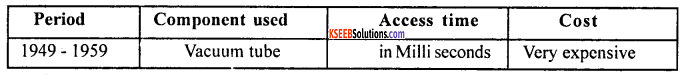The first computers used vacuum tubes for electronic circuitry and magnetic drums for memory. They were very expensive to operate and the power consumption was high, generated a lot of heat, which was often ti e cause of malfunctions.

First generation compilers were communicated through machine language to perform operations, and they could only solve one problem at a time. Input was based on punched cards and paper tape, and output was displayed on printouts.
UNIVAC and ENIAC computers are examples of first-generation computing devices.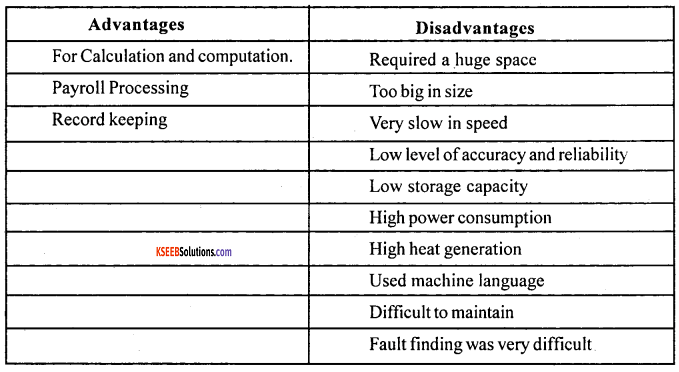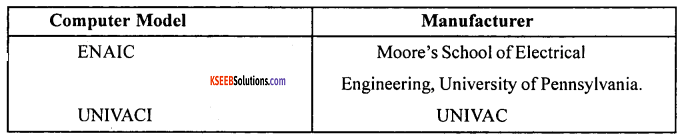Question 28.
How do you form 1’s complement of a binary number? Subtract (26)10 from (56)10 using 1’s complement subtraction method.
The 1’s complement of a number is formed by changing all 1’s to 0’s and all 0’s to 1’s.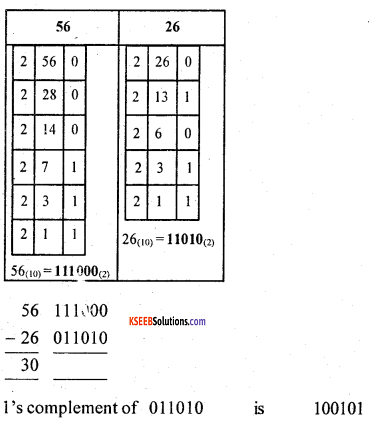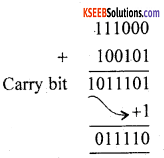Question 29.
Write a flow chart to find the factorial of a number.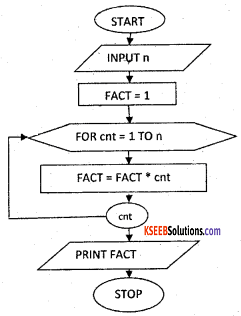Question 30.
Write the general structure of C++ program and explain any three components.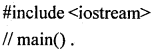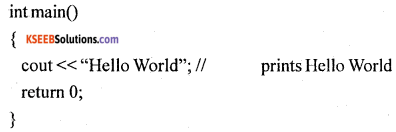The various parts of the above program:
headers, which contain information that is either necessary or useful to program. For this program, the header <iostrcam> is needed.

1. The next line // main() is where the program execution begins. It is a single-line comment available in C++. Single-line comments begin with // and stop at the end of the line.

2. The line int main() is the main function where program execution begins.

3. The pair of {} indicates the body of the main function.

4. The next line cout<< “Hello World.”; causes the message “Hello World” to be displayed on the screen.

5. The next line return 0; terminates main( )function and causes it to return the value 0 to the calling process.Question 31.
Explain Nested if-else-if statement general format with suitable example.
The ‘if else if’ statement is an extension of the “if else” conditional branching statement. When the expression in the “if’ condition is “false” another “if else” construct is used to execute a set of statements based on the expression.
Syntax:
– if(expression)
{ statements } else if(expression)
{ statements } else if(expression)
{ statements }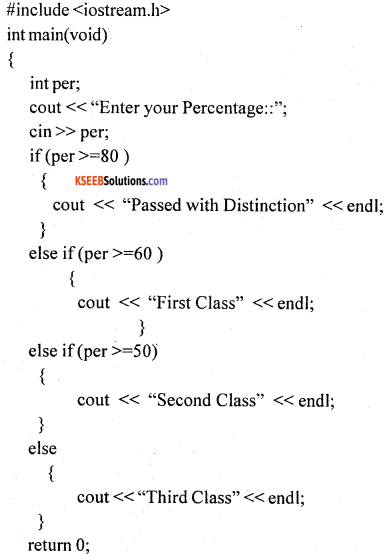}
Result:
First Class
In the above example, the ‘if else if’ statement is used to check multiple conditions based on the percentage of marks got, as input. The user entered percentage as 60, then first condition evaluated and returned with false. This resulted in checking for second if condition and resulted in true which give the print “first class”.

Question 32.
Write an algorithm to find the area and circumference of a circle.
Step 1: Start
Step 2: input r
Step 3: let pi = 3.14
Step 4: area = pi * r * r
Step 5: circum = 2 * pi * r
Step 6: print area, circum ,
Step 7: stop

Question 33.
Write a program segment to read and write the elements of one dimensional array of size N.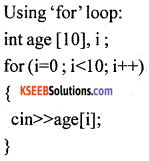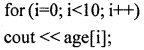Question 34.
Explain the structure of a function with an example.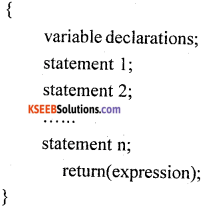1. Function type:
The function-type identifies the type of return value, which will be sent back after the function has performed its task.
Eg:
function type may be a int, float, char, void.

2. Function_name:
Function name followed by a pair of open and close parentheses ‘()’ helps to identify name of the function and its arguments. () The parentheses can contain an optional list of arguments that are passed to the function. Arguments are used to transfer/copy (pass) the values from calling function to called function.

3. Body of the function:
It is enclosed within braces. A function should have at least one executable statement and rest of the things are same as in main( ) function. The body of the function can also have an optional declaration for the variables to be used by the function only also called local variables.

4. Return(expression):
The return statement can return the value of any expression. The return statement can contain an optional expression or value that is to be returned back to the calling function.Question 35.
Write any five formatting options in ESS.
It is a toolbar that has the icons that provide the various formatting settings for cells, columns, rows, and data in Excel. For example, it can allow you to change the colours of rows, cells, and columns. It allows you to change fonts. It allows you to set the alignment for data in cells.

It allows you apply borders to cells. It allows you to change the format of numbers, like adjusting the amount of decimals they have or changing them to percentage format. All of these and other things can be done with it.

1. Format Cells Dialog Box:
For a complete list of formatting options, right-click on the highlighted cells and choose Format Cells from the shortcut menu or select Format > Cells from the menu bar.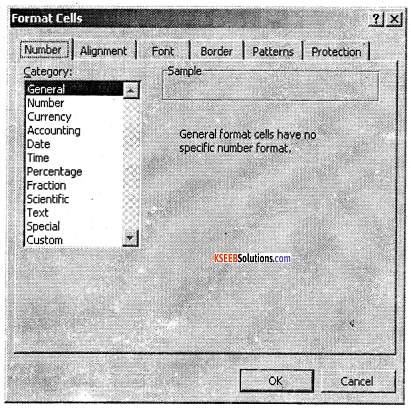2. Number tab:
The data type can be selected from the options on this tab. Select General if the cell contains text and numbers, or a different numerical category if the cell is a number that will be included in functions or formulas. The number tab can be used to limit the number decimal places.

3. Alignment tab:
These options allow you to change the position and alignment of the data within the cell. For example, right, left, center, vertical alignments, text wrap, etc.,

4. Font tab:
All of the font attributes are displayed in this tab including font face, size, style, and effects. For example, bold, italicize, underline, increase/decrease the font size, changing the color of font, fill, etc.,

5. Border and Pattern tabs:
These tabs allow us to add borders, shading, and background colors to a cell.

Question 36.
Write any five applications of ESS.
The different uses of spreadsheets are as follows:

• Performing basic mathematical operations such as adding columns and rows of figures.
• Finding values such as profit or loss.
• Calculating repayment plans for loans or mortgages.
• Finding the average, maximum, or minimum values in a specified range of data.
• Graphing or charting data, to assist users in identifying data trends.
• Sorting and filtering data to find specific information.
• Creation of simple lists and tables of alphabetic or numerical data.
• Creation and manipulation of simple (flat-file) databases.
• Establish relationships between sets of numerical data.
• Applies arithmetical, mathematical or statistical functions to numerical datasets.
• Represents datasets in graphical or chart form.

Question 37.
What is e-mail? Write its advantages.